# Doubly Linked List in Data Structures

Blog Author

Tekslate

Published Date

21st September, 2018

Ratings

Views

927

In a single linked list, it is possible to move only in the direction of links, that is we have been restricted to traversing linked list in only one direction. In the linked lists, each node provides information about where is the next node in the list. It has no knowledge about where the previous node lies in memory. If we are at say the 15th node in the list, then to reach the 14th node we have to traverse the list right from the first node. To avoid this we can store in each node not only the address of next node but also. The address of the previous node in the linked list this arrangement is often known as ‘Doubly linked list’ in data structures. The list can be traverse in either a forward or backward direction. Each node in doubly linked list must contain two link fields instead of one. Here a node has at least three fields say data, left link and right link.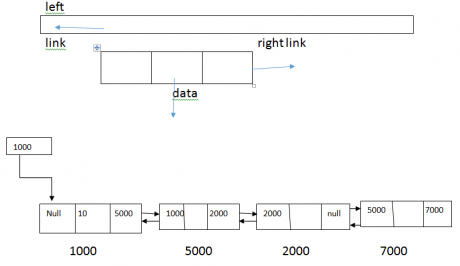### Insertion Operation

• Insertion of first Node

ptr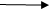bl=cur; curfl =ptr; rur=cur;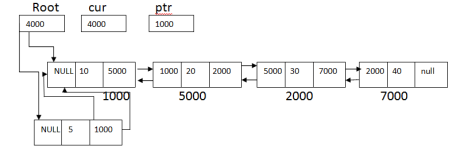1. ### Insertion after a given node:

curfl =ptr àfl; curbl =ptr; ptrflbl=cur; ptrfl =cur;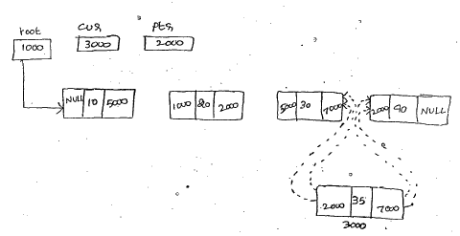## Deletion Operation

1. ### Deleting First Node

ptrflbl= ptrbl; root =ptrfl;1. ### Deleting a specified node:

ptrflbl = ptrbl; ptrblfl= ptrfl;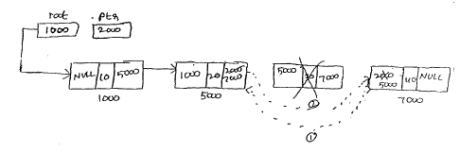### Program

Implementation of Doubly linked list #include <stdio.h> #include<conio.h> # include<stdlib.h> struct dlist { int data; struct dlist ,*fl,*bl; }; typedef struct dlist node; node *ptr,*root,*cur,*last; void display() { ptr=root; last=NULL; printf(“The list is \n”); while(ptr!=NULL) { printf(“%d”,ptrdata); if(ptrflNULL); last=ptr; ptr=ptr-fl; } printf(“\n reverse list”); ptr=last; while(ptr!=Null) { printf(“%d”,ptrdata);               ptr=ptrbl; } } void insertion() { int ele; char ch; ptr=root; cur=(node *) malloc*(sizeof(nodes)); printf(“enter data for node” ); scanf(“%d”, &curdata); curfl=curbl=NULL; ffush(stdin); printf(“do you want to insert first(Y/N):”); scanf(“%c”,&ch); if(ch==’Y’ || ch==’Y’) { ptrbl=cur; curfl=ptr; root=cur; } Else         { printf(“After which element you want to insert:”); scanf(“%d”,&ele); while(ptr!=NULL) { if(ptrdata== ele) { curfl=ptrfl; curbl=ptr; ptrflbl=cur; ptrfl=cur; break; } else ptr=ptrfl; } void deletion() { int ele; char ch; ptr=root;           fflush(stdin); printf(“Do you want to delete first(y/n)”); scanf(“%c”, &ch); if(ch==’ Y’||ch=’ Y’) { ptrflbl=ptrbl; root=ptrfl; } else { printf(“which element you want to delete :”); scanf(“%d”, &ele); while(ptr!=NULL) { if(ptrdata==ele) { ptrflbl=ptr -bl; ptrblfl=ptrfl; }                 else ptr=ptrfl; } } } void main() { int a,n; root=NULL; printf(“Enter data for node(-1)”); scanf(“%d”,&a); while(a!=-1) { cur=(node*) malloc(sizeof(nodes)); cur-> data =a; curfl=curbl=NULL; if(root==NULL) root=cur; else             { curbl=ptr; ptrfl=cur; } ptr=cur; printf(“enter data for node(-1)”); scanf(“%d”,&a); } printf(“1.display\n”); printf(“2.Insertion\n”); printf(“3. deletion \n”); printf(“4. Exit \n”); do { printf(“Enter four option :”); scanf(“%d”,&n); switch(n) { case 1: display();break; case 2: insertion();break; case 3: deletion(); break; case 4: exit(0); break; } } while(n<=4); getch(); }